Topics: AI | Data Science | Data Visualization | Deep Learning | Machine Learning | NLP | Python | R | Statistics

KDnuggets Home » News » 2018 » Jan » Tutorials, Overviews » Data Structures Related to Machine Learning Algorithms ( 18:n05 )

#Data Structures Related to Machine Learning Algorithms

If you want to solve some real-world problems and design a cool product or algorithm, then having machine learning skills is not enough. You would need good working knowledge of data structures.comments

By Peter Mills, StatsbotIllustration source

The Statsbot team has invited Peter Mills to tell you about data structures for machine learning approaches.

So you’ve decided to move beyond canned algorithms and start to code your own machine learning methods. Maybe you’ve got an idea for a cool new way of clustering data, or maybe you are frustrated by the limitations in your favorite statistical classification package.

In either case, the better your knowledge of data structures and algorithms, the easier time you’ll have when it comes time to code up.

I don’t think the data structures used in machine learning are significantly different than those used in other areas of software development. Because of the size and difficulty of many of the problems, however, having a really solid handle on the basics is essential.

Also, because machine learning is a very mathematical field, one should have in mind how data structures can be used to solve mathematical problems and as mathematical objects in their own right.

There are two ways to classify data structures: by their implementation and by their operation.

By implementation, I mean the nuts and bolts of how they are programmed and the actual storage patterns. How they look on the outside is less important than what’s going on under the hood. For data structures classed by operation or abstract data types, it is the opposite: their external appearance and operation is more important than how they are implemented, and in fact they can usually be implemented using a number of different internal representations.

### Array

I’m not kidding when I say that the basic array is the most important data structure in machine learning, and there is more to this bread-and-butter type than you might think. Arrays are important because they are used in linear algebra: the most useful and powerful mathematical tool at your disposal.

Therefore, the most common types will be the one- and two-dimensional variety, corresponding to vectors and matrices respectively, but you will occasionally encounter three- or four-dimensional arrays either for higher ranked tensors or to group examples of the former.

When doing matrix arithmetic, you will have to choose from a dizzying variety of libraries, data types, and even languages. Many scientific programming languages such as Matlab, Interactive Data Language (IDL), and Python with the Numpy extension are designed primarily for working with vectors and matrices.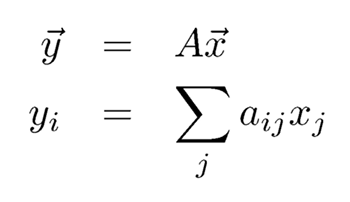But the nice thing about these data structures is that, even in more general-purpose programming languages, implementing vectors and matrices is straightforward right next to the metal, assuming the language has any Fortran DNA in it at all. Consider the translation of matrix-vector multiplication:
into C++:

```for (int i=0; i<n; i++) {
y[i]=0;
for (int j=0; j<n; j++) y[i]+=a[i][j]*x[j]
}```

### Extensible array

In most cases, arrays can be allocated to a fixed size at run time, or you can calculate a reliable upper bound. In those cases where you need your arrays to expand indefinitely, you can use an extensible array such as the vector class in the C++ standard template library (STL). Regular arrays in Matlab are similarly extensible, and extensible arrays are the basis of the entire Python language.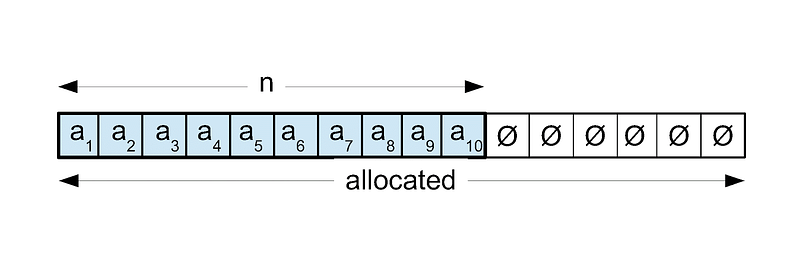In this data structure, there are two pieces of “meta-data” stored alongside the actual data values. These are the amount of storage space allocated to the data structure and the actual size of the array. As soon as the size of the array exceeds the storage space, a new space is allocated that’s twice the size, the values copied into it, and the old array deleted.

This is an O(n) operation, where n is the size of the array, but since it only happens occasionally, time to add a new value onto the end actually amortizes to constant time, O(1). It is a very flexible data structure with fast average insertions and fast access.

Extensible arrays are excellent for composing other, more complex data structures and making them extensible. For example, to store a sparse matrix: any number of new elements can be added onto the end and they are then sorted by position to make location faster. More on this later.

Sparse matrix can be used in text classification problems.

A linked list consists of several separately allocated nodes. Each node contains a data value plus a pointer to the next node in the list. Insertions, at constant time, are very efficient, but accessing a value is slow and often requires scanning through much of the list.

Linked lists are easy to splice together and split apart. There are many variations: for instance, insertions can be done at either the head or the tail; the list can be doubly-linked and there are many similar data structures based on the same principle such as the binary tree, below.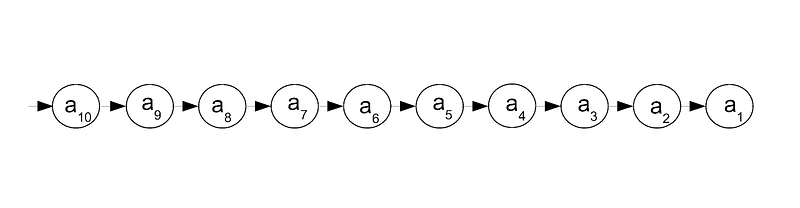Mainly, I find linked lists useful for parsing lists of indeterminate length. Afterwards, they can be converted to fixed-length arrays for fast access. For this reason, I use a linked list class that includes a method for conversion to an array.

### Binary tree

A binary tree is similar to a linked list except that each node has two pointers to subsequent nodes instead of just one. The value in the left child is always less than the value in the parent node, which in turn is smaller than that of the right child. Thus, data in binary trees is automatically sorted. Both insertion and access are efficient at O(log n) on average. Like linked lists, they are easy to transform into arrays and this is the basis for a tree-sort.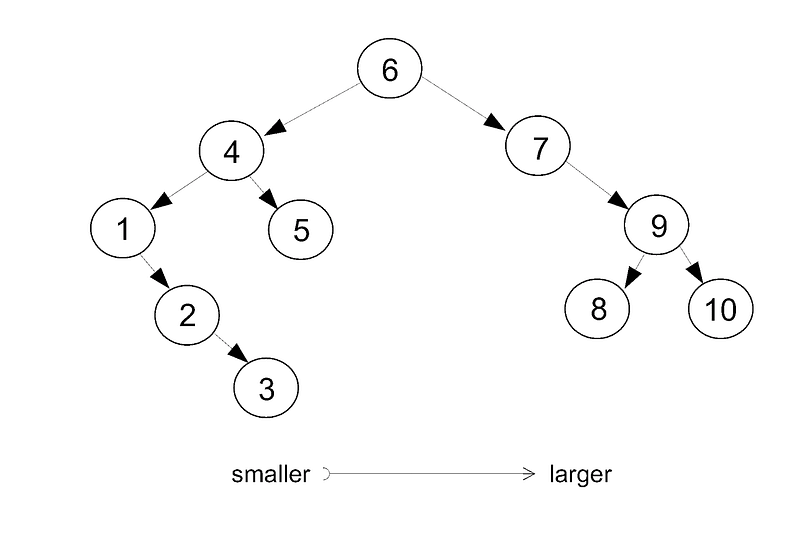### Balanced tree

If the data is already already sorted, binary trees are less efficient at O(n) worst case since the data will be laid out linearly as if it were a linked list. While the ordering in a binary tree is constrained, it is by no means unique and the same list can be arranged in many different configurations depending on the order in which it is inserted.

There are several transformations that can be applied to a tree in order to make it more balanced. Self-balancing trees perform these operations automatically in order to keep access and insertion at an optimal average.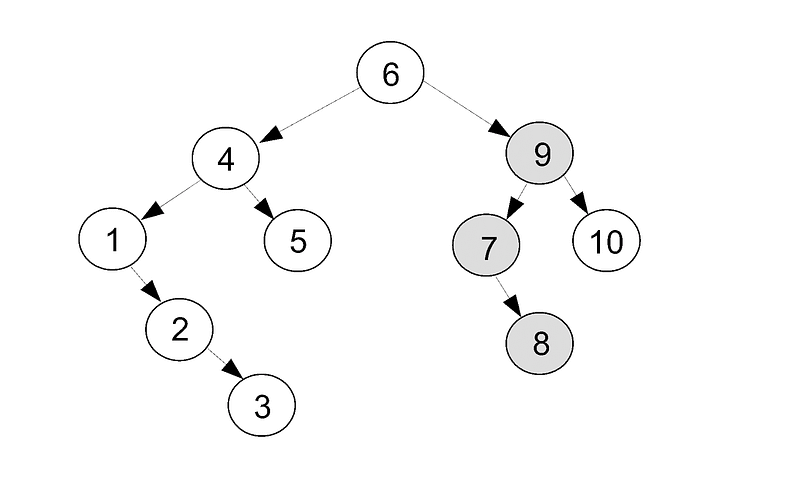A widespread problem in machine learning is finding the nearest neighbor to a particular point. This problem is needed for NN algorithm. KD tree, a type of binary tree, provides an efficient solution for that.

Top Stories Past 30 Days
Most Popular
Most SharedGet KDnuggets, a leading newsletter on AI, Data Science, and Machine Learning